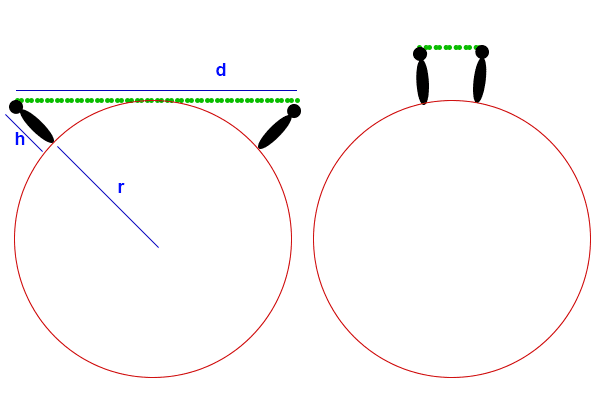# Can you fall below the horizon on an expanding balloon?

• B
• DaveC426913

#### DaveC426913

Gold Member
TL;DR Summary
Can two people lose sight of each other while standing still on an inflating balloon?
This scenario came up (as a dream) in a sci-fi novel (Robert J. Sawyer's The Terminal Man).

Two people
• of height h,
• initially within sight of each other,
• at d distance apart ,
• on a huge balloon of radius r.

As the balloon expands, they might or might not lose sight of each other over the horizon, but it seems to depend on h and initial values of r and d.

My intuition said no; they never lose sight of each other. (It could only happen in a dream where physics can be tossed aside.)

Imagine a circle inscribed in a square. As the inscribed circle and square expand the distance along the diagonal from circle to the square corner increases too.

It’s not hard to imagine that once that distance exceeds the height of both people that they lose sight of one another. Their line of sight is the side of the square and this side is tangent to the inscribed circle.

•mfb
My intuition said no
My intuition says absolutely they can lose sight of each other.

EDIT: OK, @jedishrfu beat me to it by a few secondsImagine a circle inscribed in a square. As the inscribed circle and square expand the distance along the diagonal from circle to the square corner increases too.
That's brilliant visualizing!

But yes, I'd forgotten to examine a sufficiently diverse range of values for d, h and r.

So it's true that they'd lose sight when d is comparable to r. But it would be a different story when d is << than r.So there is still a relationship between h, d and r that determines when - and if - it happens.

Last edited:
•jedishrfu
Is there a tool I can use to explore this? I suspect Wolfram Alpha can but I have never learned how to use it.

That's brilliant visualizing!

But yes, I'd forgotten to examine a sufficiently diverse range of values for d, h and r.

So it's true that they'd lose sight when d is comparable to r. But it would be a different story when d is << than r.
View attachment 288828

So there is still a relationship between h, d and r that determines when - and if - it happens.
Yes, there is a relationship. One can work it out with pencil, paper and algebra.

In the above picture you are using ##d## as the linear distance from head to head. We can use this to compute ##\theta##. For convenience, we will make ##\theta## the half-angle between the two people. Naturally, this angle will be preserved as the balloon inflates or deflates.$$\theta=\sin^{-1}\frac{d}{2(r_0+h)}$$The critical point for line of sight is when $$(r+h)\cos \theta = r$$We want to solve for the radius at which this occurs for a given ##h## and ##\theta##. So we subtract ##r \cos \theta## from both sides, yielding:$$h \cos \theta = r(1-\cos \theta)$$Now we can divide both sides by ##1-\cos \theta## yielding:$$h\frac{\cos \theta}{1-\cos \theta} = r$$

If the two guys start out directly opposite from one another then they can never establish a line of sight. The cosine of the half angle is exactly one and the formula fails on a divide by zero. Otherwise, there is always a value of r low enough to establish line of sight or high enough to break it.

••mfb and DaveC426913
If the two guys start out directly opposite from one another then they can never establish a line of sight. The cosine of the half angle is exactly one and the formula fails on a divide by zero. Otherwise, there is always a value of r low enough to establish line of sight or high enough to break it.
Cool. This was what I was groping for.

I was thinking of drawing a graph with d as one axis, and r as the other, where they are ratios of the unit h.

You can show that it depends on the radius purely from dimensional analysis. For any given separation angle and radius there is a minimal height to see each other. That minimal height has to be proportional to the radius, the only length scale in the system. This means h=f(θ)r for the boundary, or r<h/f(θ) for visibility. The explicit calculation then produces the angular dependence f(θ).

•jbriggs444